# Automorphic function

(diff) ← Older revision | Latest revision (diff) | Newer revision → (diff)

A meromorphic function of several complex variables that is invariant under some discrete group of transformationsof analytic transformations of a given complex manifold: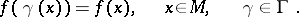Automorphic functions are often defined so as to include only functions defined on a bounded connected domainof the-dimensional complex spacethat are invariant under a discrete groupof automorphisms of this domain.

The quotient space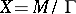can be given a complex structure and automorphic functions are then meromorphic functions on. A large number of cases studied concern a spacewith a compactification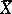. It is then natural to include in the definition of an automorphic function the requirement that it can be extended to the entire spaceas a meromorphic function. If(i.e.is a bounded connected domain), this condition must be required foronly (ifor ifis compact, the condition is automatically fulfilled). It can readily be shown that the automorphic functions constitute a field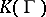and the study of this field is one of the main tasks in the theory of automorphic functions.

Automorphic functions of a single variable have been very thoroughly studied. The theoretical foundations were laid by F. Klein  and H. Poincaré  in the 19th century. The manifoldusually considered at that time is a simply-connected domain. Three cases are distinguished: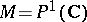(the complex projective line, or the Riemann sphere),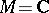and(the upper half-plane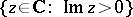). In the first case the discrete groupsare finite, the curvesare algebraic curves of genus 0 (cf. Genus of a curve) and, consequently, the automorphic functions generate a field of rational functions. Examples of automorphic functions in the caseare periodic functions (thus, the function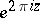is invariant under the translation group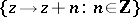) and, in particular, elliptic functions. In this latter case, the curveis compact and is an elliptic curve, while the fieldis the field of all algebraic functions on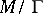. Finally, forand a discrete groupsuch thatis compact or has a finite volume (in the Poincaré metric),is an algebraic curve andis again the field of algebraic functions on. The genus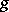of this curve may be determined by constructing a fundamental domain forin the form of a polygon in the upper half-plane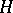(here regarded as the Lobachevskii plane). The basic method for constructing an automorphic function in this situation is to consider the quotient of two automorphic forms (cf. Automorphic form) of the same, sufficiently large, weight. The method is due to Poincaré, who used it to prove the results mentioned above concerning the structure of the fields of automorphic functions , , . An analogous construction for elliptic functions is to represent such functions in terms of quotients of theta-functions. It can be shown, using uniformization theory, that all fields of algebraic functions of a single variable are obtained in this way .

These results, which were obtained as early as the 19th century, give a full description of the fields of automorphic functions forand of the groupssuch that the space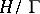has finite volume. The case of groupsfor whichhas infinite volume (Kleinian groups) is much more difficult; the problems involved are still being intensively investigated , .

In the 20th century the theory of automorphic functions concentrates on functions of several variables. Perhaps the only case of automorphic functions ofvariables studied in detail in the 19th century concerned Abelian functions, which are related to Abelian varieties in a way similar to the relationship between elliptic functions and elliptic curves , . The first example of automorphic functions ofvariables on a bounded domainare the modular functions of C.L. Siegel  (cf. Modular group). Their domain of definition is an-dimensional generalization of the upper half-plane, and is one of the main examples of a bounded, symmetric domain. Siegel must also be credited with the first general results obtained concerning arbitrary automorphic functions on a bounded domain. He generalized Poincaré's construction of automorphic functions, mentioned above, and showed that the fieldalways contains at leastalgebraically independent functions.

Subsequent efforts were aimed at exhibiting domainsand groupsfor which the following theorem on algebraic relations is true. If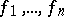are algebraically independent automorphic functions, then the fieldis a finite algebraic extension of the field of rational functions.

At the time of writing (1977) this theorem was proved for the following cases: 1) if the quotient space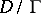is compact ; 2) if the groupis pseudo-concave ; and 3) ifis a symmetric domain andis an arithmetic group. A pseudo-concave group is defined as follows. Letbe a subdomain of a domainwith closure also contained in. In this situation a boundary point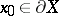is said to be pseudo-concave if for any open neighbourhood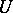ofand for any function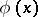regular inthere exists a pointfor which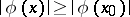. A groupis said to be pseudo-concave if there exists a subdomain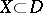such that each boundary point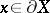can be transformed by means of an element ofinto an interior point ofor into a pseudo-concave point of the boundary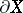.

The nature and the properties of the algebraic varieties occurring in the theory of automorphic functions ofvariables have not been intensively studied, as distinct from the case of a single variable.

Important generalizations of the concept of automorphic functions — automorphic forms, theta-functions (cf. Theta-function) and certain other generalizations — are all special cases of the following general construction. Consider a fibre bundle (cf. Fibration)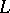overand an action of a groupon it. It is then possible to consider the sections ofthat are invariant under. An automorphic function is obtained if the fibre bundleand the action of the groupare both trivial.

The study of automorphic functions revealed the important role played by the group of automorphisms of a domain. It is in this way that the concepts and methods of the theory of automorphic functions were applied in the theory of algebraic groups, in which they play an important part in the description of infinite-dimensional representations .

From the very beginning of its development, the theory of automorphic functions has been connected in numerous ways with other branches of mathematics. This applies in particular to algebraic geometry. In addition to the results discussed above, methods in the theory of automorphic functions are important in the study of moduli varieties for objects such as algebraic curves and Abelian varieties. Automorphic functions are also of importance in number theory. At the time of writing they are the only tool in the study of zeta-functions of algebraic varieties . Another very promising number-theoretical direction in the theory of automorphic functions is the study of-adic automorphic functions and forms . Finally, one must mention the application of automorphic functions to the study of ordinary differential equations in a complex domain  and in the construction of solutions of algebraic equations of degrees higher than four.

How to Cite This Entry:
Automorphic function. Encyclopedia of Mathematics. URL: http://encyclopediaofmath.org/index.php?title=Automorphic_function&oldid=11477
This article was adapted from an original article by A.N. AndrianovA.N. Parshin (originator), which appeared in Encyclopedia of Mathematics - ISBN 1402006098. See original article